Type Here to Get Search Results !

# MATHEMATICS FORM FIVE TOPIC 8: LINEAR PROGRAMING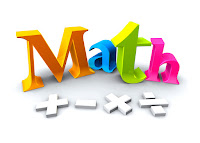## LINEAR PROGRAMING

Is a branch of mathematics which enables one to solve problems which either the greatest or minimum/least value of a certain quantity is required under some given limitations or constraints.

Example

In a big – organization, decision about distribution in order to realize maximum profit or reduce costs of production are done by use of linear programming.

Limitation/ constraints are translated by linear inequalities.

Greatest value or least value will be expressed as a function (called the objective function)

Introduction

Drawing of linear inequalities

Example 01

Draw and show the half plane represented by 8x +2y ≥16

Solution

For 8x +2y ≥16; draw 8x + 2y = 16

For x – intercept, y = 0

8x = 16

X = 2

For y – intercept, x = 0

2y = 16

Y = 8

Using (0, 0) as a test point

8(0) +2(0) ≥ 16

0 ≥ 16 (False)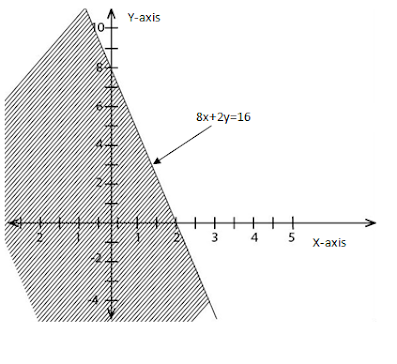Example 02

Determine the solution set of the simultaneous inequalities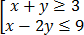Solution

x + y ≥ 3 draw x + y = 3 (full line)

x + y = 3

At x – intercept y = 0 at y – intercept x = 0

x = 3 y = 3

For x – 2y ≤ 9, x – 2y = 9

At x – intercept y = 0 at y – intercept, x = 0

X =9 -2y = 9

Y =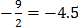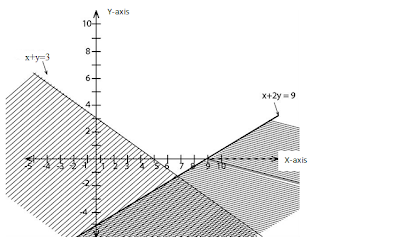Using (0, 0) as a test point Using (0,0) as a test point

x + y ≥ 3 x – 2y ≤ 9

0 + 0 ≥ 3 0 – (2)(0) ≤ 9

0 ≥ 3 (F) 0 ≤ 9 (T)

The clear part is the solution set

The solution set is called the feasible region

Questions

Draw by shading unwanted regions of the half planes represented by following simultaneous inequalities

(i) y≥ 2x – 1, y ≤ -1

(ii) y≤ 2x – 1, y ≥ x – 3, y ≥ -1

(iii) y < 2x – 1, y ≤ -1

(iv) 6x + 9y ≥ 12

0.4x + 0.1y ≥ 0.2

32x + 10y ≥ 20

Evaluation of a function satisfied by the given set of inequalities

→Example

Find the maximum and minimum value of c = 4x + 3y + 38 subjected to

x + y ≥ 5

0 ≤ y ≤ 6 0 ≤ x ≤ 5

x ≥ 0, y ≥ 0

Solution

For x + y ≥ 5

x + y = 5

When x = 0, y = 5

y = 0, x = 5

For 0 ≤ y ≤ 6

0 = y = 6

Line y = 6

For 0 ≤ x ≤ 5

O = x = 5

Line x = 5

Test points x ≥ 0, shade left of x = 0

(0, 0) y ≥ 0, shade below of x = axis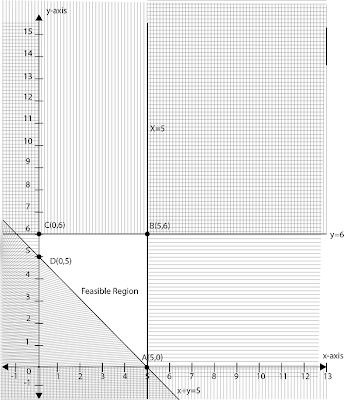: . The maximum value of c = 76 and occurs at (5, 6)

The minimum value of c = 53 and occurs at (0, 5)

Questions

1. Find the maximum and minimum values of the given functions and the value x and y where they occur

(i) Z = 4x + 3y

Subject to

x + 2y ≤ 10

3x + y ≤ 5

x ≥ 0, y ≥ 0

(ii) P = 134x + 20y

Subject to

x + y ≤ 160

10 ≤ x ≤ 60

0 ≤ y ≤ 120

(iii) T = 4x +7y

Subject to.

x + y ≤ 18

5 ≤ x ≤ 10

3 ≤ y ≤ 10

x ≥ 0, y ≥ 0

(iv) P = 2x + 4y

Subject to

2x + 3y ≥ 3

-5x + 4y ≤ 0

3x + 4y ≤ 18

X ≥ 0, y ≥ 0.

FORMULATION OF A LINEAR PROGRAMMING PROBLEM.

Steps in formulating a linear programming problem.

1. * Read the problem several times and assess what is known and what is to be determined.

2. * Identify the unknown quantities and assign variables to them, be careful about the units.

3. * Determine the objective function; it involves the quantity to be maximized or minimized.

4. * Translate the constraints into linear inequalities,

* Constraints are limitation or restrictions to the problem for each constraints the units must be same.

5. * Graph the constraints and find the feasible solution

6. * Find the corner points of the feasible solution. These are points of intersection of the graph

7. * Evaluate the objective function. Highest value of the objective function has to be maximized or smallest value to be minimized.

Example

A student has 120 shillings to spend on exercise books. At a school shop an exercise book costs 8 shillings, at stationery store an exercise book costs 12 shillings. The school has only 6 exercise books and the student wants to obtain the greatest number of exercise books possible using the money. Find the greatest number of exercise books he can buy.

Solution

Let x be number of exercise books to be bought at school shop

Let y be number of exercise books to be bought at stationery shop

Objective function

Let f (x, y) = objective function

Then f (x, y) = x + y

→Constrains or linear inequalities

8x + 12y ≤ 120

x ≤ 6

Non – Negative constraints

x ≥ 0

y ≥ 0

→Equations

8x + 12y = 120

4x + 6y = 60

2x + 3y = 30

When x = 0, y = 10

y = 0, x = 15

x = 6, y = 0

x = 6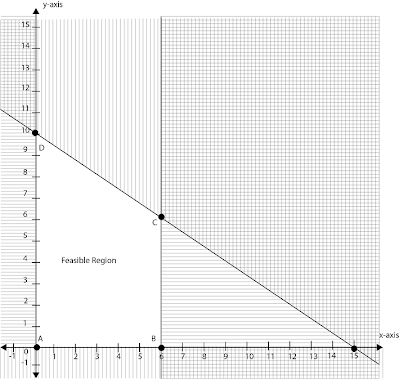Corner points C = 4x + 3y + 38 A (5, 0) 4 (5) + 3 (0) +38 = 58 B (5, 6) 4 (5) + 3 (6) + 38 = 76 C (0, 6) 4 (0) + 3 (6) +38 = 56 D (0, 5) 4 (0) + 3 (5) + 38 = 53

: . The greatest numbers of exercise books he can buy are 12 books 6 from the school shop and 6 from stationery.

Example

Student in a certain class are about to take a certain test of BAM which has two sections A and B; where in section A each question worth 10 marks while in section B; each worth 25 marks. The student must do at least 3 questions of section A; but not more than 12. A student must also do 4 questions from section B but not more than 15. In addition students cannot do more than 20 questions. How many questions of each type should the student do to obtain the maximum scores?

Solution

Let x be number of questions to be done in section A

Let y be number of questions to be done in section B

→Objective function

f (x, y) = 10x + 25y

→Constrains

3 ≤ x ≤ 12

4 ≤ y ≤ 15

x + y ≤ 20

x ≥ 0, y ≥ 0Maximize f (x, y) = 10x + 25y subject to;

3 ≤ x ≤ 12

4 ≤ y ≤ 15

x + y ≤ 20

x ≥ 0, y ≥ 0

Equations

3 = x = 12

4 = y = 15

x + y = 20

x = 20, y = 0

x = 0, y = 20

x = 0

y = 0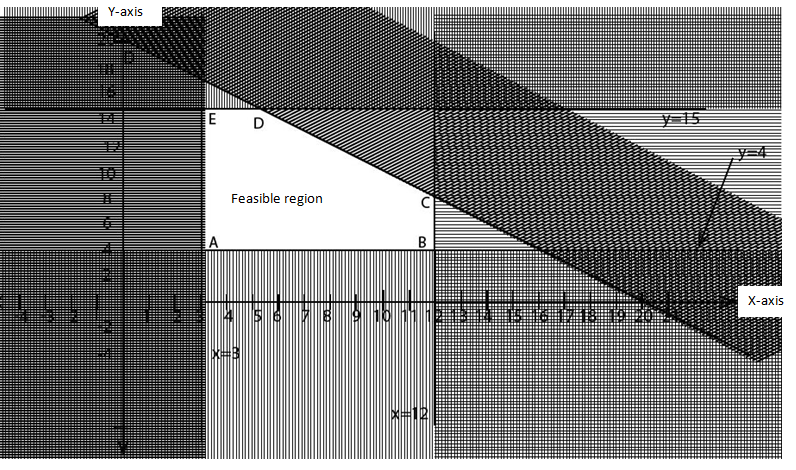Corner points F (x, y) = x + y A (0, 0) 0 + 0 = 0 B (6, 0) 6 + 0 = 6 C ( 6, 6) 6 + 6 = 12 D (0, 10) 0+10 = 10The student should do 5 questions from section A and 15 questions from section B to obtain maximum score of 425.

Diet problems on linear programming problem

Example 01

A doctor prescribes a special diet for patients containing the following number of units of Vitamin A and B per kg of two types of food F1 and F2

 Corner Points f(x, y) = 10x + 25y A (3, 4) 10 (3) + 25 (4) = 130 B (12, 4) 10 (12) + 25 (4) = 220 C (12, 8 ) 10 (12) + 25 (8) = 320 D (5, 15) 10 (5) + 25 (15) = 425 E (3, 15) 10 (3) + 25 (15) = 405

If the minimum daily intake required is 120 units of A and 70 units of B, what is the least total mass of food a patient must have so as to have enough of these vitamins?

EXAMPLE 02

Rice and beans provide maximum levels of protein, calories and vitamin B2. If used as a staple diet. The food values per kg of uncooked rice and beans are as shown in the table below.

 Type of Food Vitamin A Vitamin B F1 20 units/kg 7 units/kg F2 15 units /kg 14 units /kg

What is the lowest cost of diet meeting, these specifications?

Solution.

Let x be number of kg of rice to be bought

Let y be number of kg of beans to be bought

→Objective function

400x + 500y

Constrains

60x + 90y ≤ 120

3200x + 1000y ≤ 2000

0.4x + 0.1y ≤ 0.2Minimize f (x, y) = 400x + 500y Subject to;

60x + 90y ≤ 120

3200x + 1000y ≤ 2000

0.4x + 0.1y ≤ 0.2

x ≥ 0, y ≥ 0

For 60x + 90y ≥ 120

60x + 90y = 120

2x + 3y = 4

When x = 0, y = 1.3

Y = 0, x = 2

For 3200x + 1000y ≥ 2000

32x + 10y = 20

16x + 5y = 10

When x = 0, y = 2

Y = 0, x = 0.63

For 0.4x + 0.1y ≤ 0.2

0.4x + 0.1y = 0.2

When x = 0, y = 2

When y = 0, x = 0.5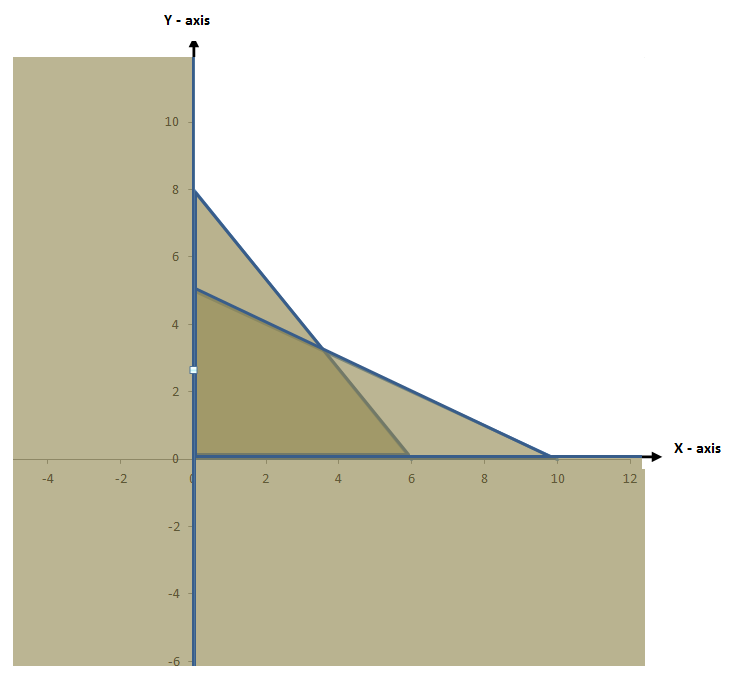Protein /kg Calories/kg Vitamin B2/kg Price kg Rice 60g 3200 cal 0.4 400 Beans 90g 1000 cal 0.1 500 Min daily req. 120 2000 cal 0.2

: . The least total mass a patient should have is 6.8kg i.e. 3.6kg of food 1 and 3.2 kg of food 2.

Question

1. A doctor prescribes that in order to obtain adequate supply of vitamin A and C his patient should have portions of food 1 and food 2. The number of units of vitamin A and C are given in the following table

 Corner points F (x, y) = x + y A (0, 8) 0 + 8 = 8 B (3.6, 3.2) 3.6 + 3.2 = 6.8 C (10,0) 10 + 0 = 10

The doctor prescribes a minimum of 14 units of vitamin A and 21 units of vitamin C. What are the least portions of food 1 and food 2 that will fit the doctor’s prescriptions?

LINEAR PROGRAMMING PROBLEMS

1. Two printers N and T produce three types of books. N produces 80 types I books per day, 10 type II books per day and 20 types III books per day, while T produces 20 types I books per day 10 type II books per day and 70 types III books per day. The orders placed are 1600 type I, 500 type II and 2100 type III books. The daily operating costs for N shs. 10,000/=, for T shs, 20,000/= how many days should each printer operate to meet the orders at a minimum cost.

2. A small textile company manufactures three different size of shirts, Large (L), medium (M) and small (S) at two different plants A and B. The number of shirts of each size produced and the cost of production per day are as follows!

 A C Food 1 3 2 Food 2 1 7

(i) How many days per month should each factory operate in order to minimize total cost.

(ii) What is the minimum cost of production

Solution 01

Let x be number of days printer N should operate

Let Y be number of days printer T should operate

→Objective function (f(x, y))

10000x + 20000y

→Constrains

80x + 20y ≥ 1600

10x + 10y ≥ 500

20x + 70y ≥ 2100

X ≥ 0, y ≥ 0

→Equations

80x + 20y ≥ 1600

80x + 20y = 1600

8x + 2y = 160

When x = 0, y = 80

y = 0, x = 20

10x + 10y ≥ 500

10x + 10y = 500

x + y = 50

When x = 0, y = 50

y = 0, x = 50

20x + 70y ≥ 2100

20x + 70y = 2100

2x + 7y = 210

When x = 0, y = 30

y = 0, x = 105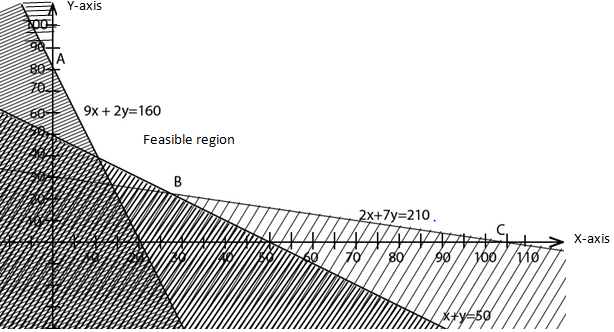A B Monthly demand Large size per day 50 60 2500 Medium size per day 100 70 3500 Small size per day 100 200 7000 Production Cost per day T. shs. 2500 3500 _Printer N should be operated for 28 days and printer T should work for 22 days to meet the orders at minimum cost.

Solution 02

Let x be number of days per month factory A should operate

Let y be number of days per month factory B should operate

→Objective function

F (x, y) = 2500x + 3500y

→Constrains

50x + 60y ≥ 2500

100x + 70y ≥ 3500

100x + 200y ≥ 7000

x ≥ 0, y ≥ 0Minimize f (x, y) = 2500x + 3500y

Subject to 50x + 60y ≥ 2500

100x + 70y ≥ 3500

100x + 200y ≥ 7000

X ≥ 0, y ≥ 0

→Equations

50x + 60y = 2500

When y = 0, x = 50

X = 0, y = 41.7

100x + 70y = 3500

When y = 0, x = 35

x = 0, y = 50

100x + 200y = 7000

When y = 0, x = 70

X = 0, y = 35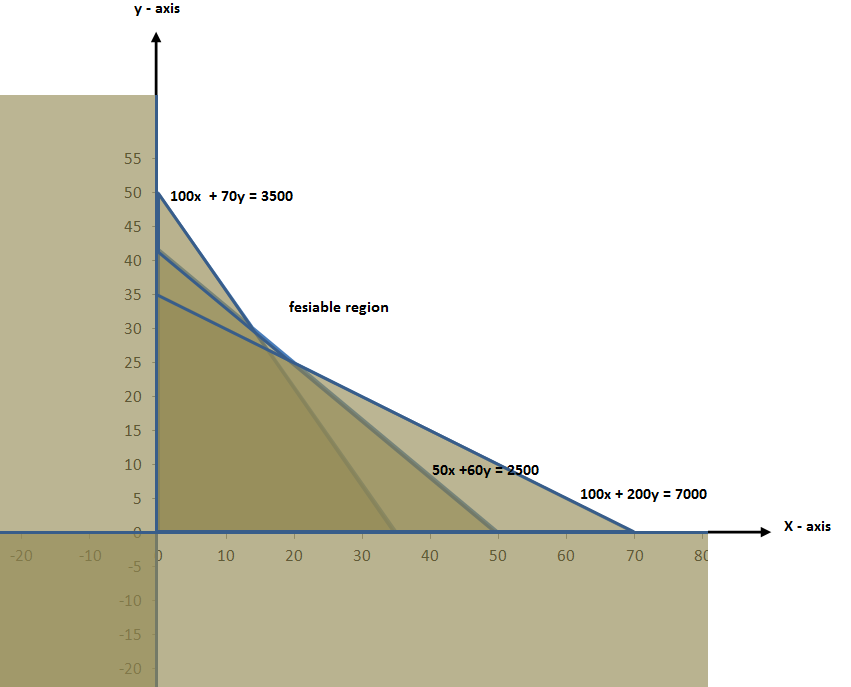Corner points F (x, y) = 10000x + 20000y A (0, 80) 10000 (0) + 20000 (80) = 1,600,000 B (28, 22) 10000 (28) + 20000 (22) = 720,000 C (105, 0) 10,000 (105) + 20000 (0) = 1,050,000Factory A should operate for 20 days and factory B should operate for 25 days in order to minimize total cost.

→Minimum cost of production is 137,500

3. In a certain garage the manager had the following facts floor space required for a saloon is 2m2 and for a lorry is 3m2. Four technicians are required to service a saloon car and three technicians for a lorry per day. He has a maximum of 24m2 of a floor space and a maximum of 36 technicians available; in addition he is not allowed to service more Lorries than saloon cars. The profit for serving a saloon car is 40,000/= and a lorry is 60,000/=. How many motor vehicles of each type should be serviced daily in order to maximize the profit?

Solution

Let x be number of saloon cars to be serviced daily

Let y be number of Lorries to be serviced daily

→Objective function

F (x, y) = 40000x + 60000y

→Constrains

2x + 3y ≤ 24

4x + 3y ≤ 36

x ≥ y

x ≥ 0 and y ≥ 0Maximize f (x, y) = 40000x + 60000y

Subject to 2x + 3y ≤ 24

4x + 3y ≤ 36

x ≥ y

x ≥ 0 and y ≥ 0

→Equations

2x + 3y ≤ 24

When x = 0, y = 8

Y = 0, x = 12

4x + 3y ≤ 36

When x = 0, y = 12

y = 0, x = 9

x = y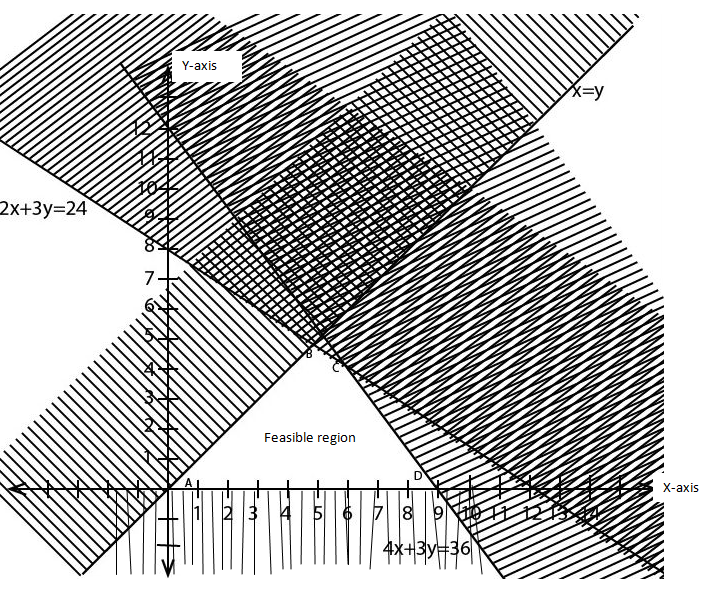Corner points F (x, y) = 2500x + 3500y A (0,50) 2500 (0) + 3500 (50) = 175,000 B (15, 30) 2500 (15) + 3500 (30) = 142,500 C (20,25) 2500 (20) + 3500 (25) = 137,500 D (70, 0) 2500 (70) + 3500 (0) = 175,0006 saloon cars and 4 Lorries should be serviced daily to maximize profit to 480,000/=

More example

A builder has two stores, one at S1 and the other at S2. He is building houses at P1, P2, and P3. He needs 5 tons of bricks at P1, 6 tons of bricks at P2 and 4 tons of bricks at P3. The stores contain 9 tons of bricks at S1 and 6 tons of bricks at S2. The transport cost per ton are shown in the diagram

 Corner points F (x, y) = 40000x + 60000y A (0, 0) 40000 (0) + 60000 (0) = 0 B (4.8, 4.8) 40000 (4.8) + 60000 (4.8) = 480,000 C (6,4) 40000 (6) + 60000 (4) = 480,000 D (9, 0) 40000 (9) + 60000 (0) = 360,000

How does the builder send his bricks at a minimum cost? What is the minimum overall cost?

Solution

Let the builder send x tons of bricks from S1 to P1 and y tons of bricks from rel="noopener" target="_blank">S1 to P2

Then the transportation of bricks to P1, P2 and P3 will be as follow: –

 To From P1 P2 P3 S1 6/= 3/= 4/= S2 4/= 2/= 6/=

The constrains are obtained as follows

x ≥ 0, y ≥ 0

9 – (x + y) ≥ 0 i.e. x + y ≤ 9

5 – x ≥ 0 i.e. x ≤ 5

6 – y ≥ 0 i.e. y ≤ 6

4 – [9 – (x + y)} ≥ 0 i.e. x + y ≥ 5

Objective function

F (x, y) = 6x + 3y + 4(9 – (x + y)) + 4 (5 – x) + 2(6 – y) +6 [4 – (9 – (x + y)]

= 6x + 3y +36 – 4x + 4y + 20 – 4x + 12 – 2y + 24 – 54 + 6x + 6y

F (x, y) = 4x – 3y + 38Minimize f (x, y) = 4x – 3y + 38

Subject to x + y ≤ 9

x + y ≥ 5

x ≤ 5, y ≥ 0

y ≤ 6, y ≥ 0

→Equation

x + y = 9

When x = 0, y = 9

y = 0, x = 9

x + y = 5

x = 0, y = 5

y = 0, x = 9

x = 5

y = 6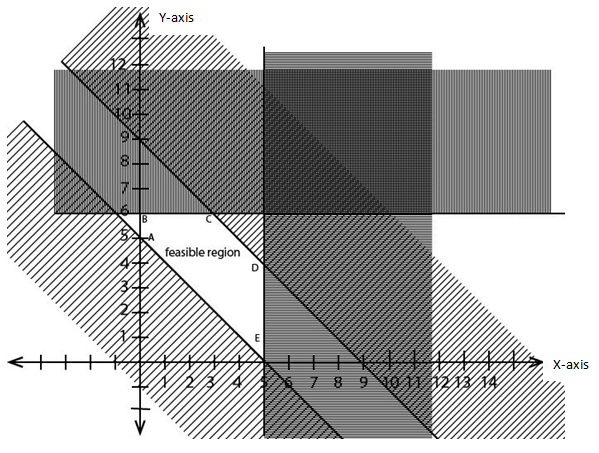To From P1 P2 P3 S1 X Y 9 – (x + y) S2 5 – x 6 – y 4 – [9 – (x + y)]The builder should send the bricks of tons as follows

 Corner points F (x, y) = 4x + 3y + 38 A (0, 5) 4 (0) + 3 (5) + 38 = 53 B (0, 6) 4 (0) + 3 (6) + 38 = 56 C (3, 6) 4 (3) + 3 (6) + 38 = 68 D (5, 4) 4 (5) + 3 (4) + 38 = 70 E (5, 0) 4 (5) + 3 (0) + 38 = 58The overall minimum cost is 53/=

EXERCISE

There is a factory located at each of the places P and Q. From these location a certain commodity is delivered to each of the three deports situated at A, B and C. The weekly requirements of the deports are respectively 5,5 and 4 unit of the commodity while the production capacity of the factories P and Q are 8 and 6 units respectively, just sufficient for requirement of deports. The cost of transportation per unit is given.

 To From P1 P2 P3 S1 5 4 S2 5 1 0

Formulate this linear programming problem and how the commodities can be transported at minimum cost. What is the overall minimum cost?

Mathematics (from Ancient Greek μάθημα; máthēma: ‘knowledge, study, learning’) is an area of knowledge that includes such topics as numbers (arithmetic, number theory), formulas and related structures (algebra), shapes and the spaces in which they are contained (geometry), and quantities and their changes (calculus and analysis).

Most mathematical activity involves the use of pure reason to discover or prove the properties of abstract objects, which consist of either abstractions from nature or—in modern mathematics—entities that are stipulated with certain properties, called axioms. A mathematical proof consists of a succession of applications of some deductive rules to already known results, including previously proved theorems, axioms and (in case of abstraction from nature) some basic properties that are considered as true starting points of the theory under consideration.

Mathematics is used in science for modeling phenomena, which then allows predictions to be made from experimental laws. The independence of mathematical truth from any experimentation implies that the accuracy of such predictions depends only on the adequacy of the model. Inaccurate predictions, rather than being caused by incorrect mathematics, imply the need to change the mathematical model used. For example, the perihelion precession of Mercury could only be explained after the emergence of Einstein’s general relativity, which replaced Newton’s law of gravitation as a better mathematical model.

But for more post and free books from our site please make sure you subscribe to our site and if you need a copy of our notes as how it is in our site contact us any time we sell them in low cost in form of PDF or WORD.

Tags